# Research

• If your institute is interested in these projects, do not hesitate to contact me, I would be happy to give a talk about them.
• If, on the other hand, you are interested in carrying out a project related to them, please contact me to discuss it.
• Kindly click on this link to directly access my list of publications. Additionally, you can click on this link to explore a potential research proposal aligned with my work.

#### [Poster session] Extending Noether’s Theorem to Nonlocal Theories

Date: July 19, 2023

Speaker: Carlos Heredia

Presentation: PDF

The exploration of global and local symmetries in the realm of convolutional neural network (CNN) architectures has emerged as an intriguing area of research. In arXiv: 2105.13926 suggest that symmetries may hold significant roles in the construction of CNN architectures, and that understanding the associated conserved quantities can provide valuable insights into the dynamics of network evolution during training. In order to accomplish this objective, the first requirement is an expansion of Noether’s theorem that applies to nonlocal theories. Hence, this poster presents a novel proof of the extension of Noether’s theorem to nonlocal Lagrangians characterized by an infinite number of degrees of freedom. By establishing this extension, we unlock new possibilities for understanding complex systems governed by nonlocal operators, such as, the convolution one. It relies on arXiv: 2304.10562, and, up until now, this extension has demonstrated successful application across various scenarios. These include p-adic particles/strings, different examples of non-local harmonic oscillators, and electrodynamics of dispersive media. In each of these cases, it has proven capable of replicating established results and, most importantly, has yielded novel results.#### [Talk]Noether’s Theorem for Nonlocal Lagrangians

Date: June 22, 2023

Speaker: Carlos Heredia

Presentation: PDF or Keynote

It is well known that the essence of Noether’s theorem is the connection between symmetries and conservation laws. For this reason, this talk proposes an extension of Noether’s theorem for nonlocal theories, focusing on nonlocal Lagrangians with a finite number of degrees of freedom. In particular, the nonlocality describing this type of Lagrangian will be given by integrodifferential operators.  Next, we will focus on invariance under time translations to define the (conserved) energy function. Moreover, using this conserved quantity obtained through this extension, we will infer a suitable definition for momenta, which could open up the possibility of developing a Hamiltonian formalism for such theories. This talk is mainly based on these three works: [arXiv] 2002.127252105.104422203.02206.#### [Ph.D. Thesis] Nonlocal Lagrangian formalism

Journal: arXiv – 2304.10562 [hep-th] (or directly here)

Date: Mar 31, 2023

Author: Carlos Heredia (supervised by Prof. Josep Llosa)

Presentation: PDF or Keynote

This thesis aims to study nonlocal Lagrangians with a finite and an infinite number of degrees of freedom. We obtain an extension of Noether’s theorem and Noether’s identities for such Lagrangians. We then set up a Hamiltonian formalism for them. Furthermore, we show that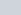-order Lagrangians can be treated as a particular case, and the expected results are recovered. Finally, the method developed is applied to different examples: nonlocal harmonic oscillator,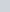-adic particle,-adic open string field, and electrodynamics of dispersive media.

#### Infinite Derivatives vs Integral Operators. The Moeller-Zwiebach paradox

Journal: arXiv – 2302.00684 [hep-th]

Date: Feb 27, 2023

Authors: Carlos Heredia and Josep Llosa

We study the relationship between integral and infinite-derivative operators. In particular, we examine the operator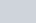that appears in the theory of-adic string fields,  as well as the Moyal product that arises in non-commutative theories. We also try to clarify the paradox raised by Moeller and Zwiebach, which highlights the discrepancy between them.

#### [Dissemination] The beauty of symmetries

Date: November 23, 2022

Speaker: Carlos Heredia

Presentation: PDF or Keynote

Symmetries (in my opinion) are the closest concept we have to describe the beauty of objects. They are everywhere, from the smallest entities, such as atoms, to the largest, such as galaxies. Finding them is synonymous (at least in physics) with simplicity. However, they can be used for much more! Thanks to the work of Emmy Noether, one of the most important, relevant, and brilliant mathematicians of the 20th century, we have a potent tool for physics to deepen our understanding of the constituents of nature. For this reason, in this talk, we shall explain (in an informative way) Noether’s theorem and how symmetries can help us to understand the nature surrounding us.

#### [Talk]Noether’s theorem for non-local theories

Date: June 07, 2022

Speaker: Carlos Heredia

Presentation: PDF or Keynote

Symmetries are the closest concept to describe our reality. It is well known that the essence of Noether’s theorem is the connection between these symmetries and the conservation laws for theories whose dynamics are derived from a variational principle. This talk presents our proposed extension of Noether’s theorem for those theories that are nonlocal. To make it as illustrative as possible, we will focus on those Lagrangians with finite degrees of freedom. We will establish the variational principle for a nonlocal Lagrangian and derive the Lagrange equations. We will introduce the concept of nonlocal kinematic space and compare it with the local case. In addition, we will discuss what conditions a nonlocal total derivative must satisfy so that the Lagrange equations vanish identically and use the conserved quantity (obtained through this extension of Noether’s theorem) to infer a suitable definition for the momenta. With this definition, we will open the possibility of building a Hamiltonian formalism for such theories. This talk is mainly based on these three works: [arXiv] 2002.127252105.104422203.02206.

#### Nonlocal Lagrangian fields: Noether’s theorem and Hamiltonian formalism

Journal: Physical Review D, 105, 126002

Date: June 01, 2022

Authors: Carlos Heredia and Josep Llosa

This article aims to study non-local Lagrangians with an infinite number of degrees of freedom. We obtain an extension of Noether’s theorem and Noether’s identities for such Lagrangians. We then set up a Hamiltonian formalism for them. In addition, we show that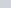-order local Lagrangians can be treated as a particular case and the standard results can be recovered. Finally, this formalism is applied to the case of-adic open string field.

#### [Talk]Noether’s theorem for non-local Lagrangian mechanics

Date: May 17, 2022

Speaker: Carlos Heredia

Presentation: PDF or Keynote

This talk aims to present the extension of Noether’s Theorem for Lagrangians with a finite number of degrees of freedom that are non-local in time. This talk is mainly based on the following works: [arXiv] 2203.022062105.10442

#### Infinite-derivative linearized gravity in convolutional form

Date: Mar 22, 2022

Authors: Carlos Heredia, Ivan Kolář, Josep Llosa, Francisco José Maldonado Torralba, and Anupam Mazumdar

This article aims to transform the infinite-order Lagrangian density for ghost-free infinite-derivative linearized gravity into non-local. To achieve it, we use the theory of generalized functions and the Fourier transform in the space of tempered distributions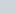. We show that the non-local operator domain is not defined on the whole functional space but on a subset of it. Moreover, we prove that these functions and their derivatives are bounded in all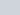and, consequently, the Riemann tensor is regular and the scalar curvature invariants do not present any spacetime singularity. Finally, we explore what conditions we need to satisfy so that the solutions of the linearized equations of motion exist in.

#### Non-local Lagrangian mechanics: Noether’s theorem and Hamiltonian formalism

Date: Sep 29, 2021

Authors: Carlos Heredia and Josep Llosa

Lagrangian systems with a finite number of degrees of freedom that are non-local in time are studied. We obtain an extension of Noether’s theorem and Noether identities to this kind of Lagrangians. A Hamiltonian formalism has then been set up for these systems. n-order local Lagrangians can be treated as a particular case of non-local ones and standard results are recovered. The method is then applied to several other cases, namely two examples of non-local oscillators and the p-adic particle.

#### Comment on «Dynamics with Infinitely Many Derivatives: The Initial Value Problem»

Date: June 8, 2021

Authors: Carlos Heredia and Josep Llosa

The definition of fractional operators usually resorts to Laplace transforms and convolution. Since the “natural” arena for the latter seems to be the space of generalised functions with support on a half-line, we pinpoint some useful notions extracted from Equations of Mathematical Physics – V. S. Vladimirov (sections from 10.2 to 10.4). Using these notions, we make some comments about the article «Dynamics with Infinitely Many Derivatives: The Initial Value Problem».

#### Energy-momentum tensor for the electromagnetic field in a dispersive medium

Date: May 10, 2021

Authors: Carlos Heredia and Josep Llosa

On the basis of a non-local Lagrangian for Maxwell equations in a dispersive medium, the energy-momentum tensor of the field is derived. We obtain the Field equations through variational methods and an extension of Noether theorem for a non-local Lagrangian is obtained as well. The electromagnetic energy-momentum tensor obtained in the general context is then specialized to the case of a field with slowly varying amplitude on a rapidly oscillating carrier.

#### [Poster session] Energy-momentum tensor for the electromagnetic field in a dispersive medium

Date: Mar 5, 2020

Speaker: Carlos Heredia

Presentation: PDF

This work aims to obtain the Electromagnetic Stress-Energy Tensor in a medium for a non-local theory. To get it, we generalize Minkowski electrodynamics to dispersive media. As a consequence of this generalization, the Lagrangian density becomes non-local due to the non-local dependencies of the magnetic permeability and electric permittivity. […] Then, we derive the field equations and, applying Noether’s theorem, the conserved energy-momentum tensor. Because non-local Lagrangians are seldom found in textbooks, we devote a non-local formalism to outline the field equations’ derivation and Noether’s theorem. […] To conclude, we study the obtained Belinfante Stress-Energy Tensor for plane wave solutions for a dispersive medium.#### [Master’s thesis] Hamilton-Jacobi formalism for non-involutive systems

Date: Jun 20, 2017

Author: Carlos Heredia (supervised by Prof. Josep Mª Pons)

This work aims to show our vision about the inconsistency of the integrability condition for non-involutive systems in the Hamilton-Jacobi formalism. A dimensional reduction is made to solve this issue, indicating that the reduced system contains the same dynamics as the original one. Moreover, to give consistency to our work, we will display this method in four examples.